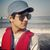# QlikView App Dev

Discussion Board for collaboration related to QlikView App Development.

Announcements
Action-Packed Learning Awaits! QlikWorld 2023. April 17 - 20 in Las Vegas: REGISTER NOW
cancel
Showing results for
Did you mean:Creator

## List Box Range Values

Hi Guys,

I need your help. Actually I am trying to do display the results on the basis of List box selection.  Following is My SQL script .

CASE

when Interval BETWEEN 0 and 6 then Less then 7 days

when Interval BETWEEN '0' and 13 then Less then 14 days

when Interval BETWEEN 0 and 27 then Less then 28 days

when Interval BETWEEN 0 and 31 then Less then 30 days

The issue with the above script is it gives wrong values. Like Less then 14 Days will give you values from  7 -13 .  not  from 0-13. same like Less then 28 days  show values between 13 and 26.

My list box (please review attached picture) given me wrong data .

Is there a way I can manage in Qlikview?

Kind Regards

Waqas Shah

Labels (3)

• ### List Box Range Values

5 RepliesSpecialist
Try this :
CASE

when Interval >=0 and Interval <= 6 then Less then 7 days

when Interval >=0 and Interval <= 13 then Less then 14 days

when Interval >=0 and Interval <= 27 then Less then 28 days

when Interval >=0 and Interval <=31 then Less then 30 daysCreator
Author

Unfortunately its not working. any other option ?Specialist

write it properly,

CASE
when Interval BETWEEN 0 and 6 then 'less then 7 days' //with ( ' ) after then
when Interval BETWEEN '0'  0 and 13 then 'Less then 14 days'
when Interval BETWEEN 0 and 27 then 'Less then 28 days'
when Interval BETWEEN 0 and 31 then 'Less then 30 days'

end as xyzCreator
Author

Sorry I did not get you . you mean trying to  add (') in SQL script ?Specialist

CASE
when Interval BETWEEN 0 and 6 then less then 7 days
when Interval BETWEEN '0' and 13 then Less then 14 days
when Interval BETWEEN 0 and 27 then Less then 28 days
when Interval BETWEEN 0 and 31 then Less then 30 days

My suggestion :

CASE
when Interval BETWEEN 0 and 6 then 'less then 7 days'
when Interval BETWEEN 0 and 13 then 'Less then 14 days'
when Interval BETWEEN 0 and 27 then 'Less then 28 days'
when Interval BETWEEN 0 and 31 then 'Less then 30 days'Community Browser